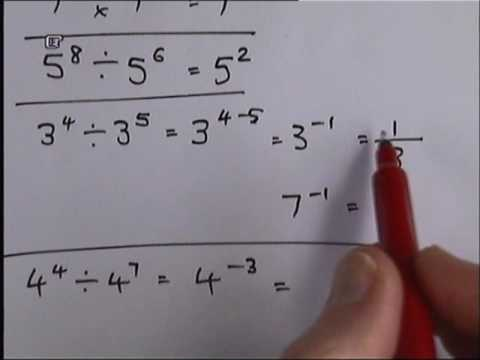## Laws of indices

Subject: General Knowledge and IQ

#### Overview

"Law of Indices" Description and Explanation with videos

### Indices are a useful way expressing large numbers in a simple way. They also provide us with many useful properties for manipulating them using what are called the Law of Indices.There are six laws of indices. They are as follows:

1. Product Law:

To multiply expressions with the same base, we copy the base and add the indices.For example,2. Quotient Law:

To divide expressions with the same base, we copy the base and subtract the indices.

For example,3. Root Law:4. Zero Law:

Any number, except 0, whose index is 0 is always equal to 1, regardless of the value of the base.

For example,5. Power Law:

To raise an expression to the nth index, we copy the base and multiply the indices.

For example,6. Negative Law:With the help of these laws, we can solve any kind of problems related to Laws of Indices.

##### Things to remember
• There are six laws of indices. They are as follows:
• - product law
• -quotient law
• -root law
• -zero law
• -power law
• -negative law
• It includes every relationship which established among the people.
• There can be more than one community in a society. Community smaller than society.
• It is a network of social relationships which cannot see or touched.
• common interests and common objectives are not necessary for society.
##### Videos for Laws of indices##### ? Index laws - Further Indices - Addition of Indices - Subtraction of Indices - Algebra